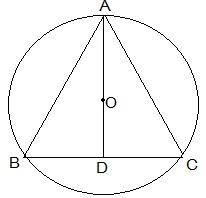Guru

# A circular park of radius 20m is situated in a colony. Three boys Ankur, Syed and David are sitting at equal distance on its boundary each having a toy telephone in his hands to talk each other. Find the length of the string of each phone. Q.6

• 3

what is the tricky way for solving the question of class 9th ncert math of Circles chapter of ncert of exercise 10.4 of math give me the best and simple way for solving this question in easy way of question no. 6 A circular park of radius 20m is situated in a colony. Three boys Ankur, Syed and David are sitting at equal distance on its boundary each having a toy telephone in his hands to talk each other. Find the length of the string of each phone.

Share

1. First, draw a diagram according to the given statements. The diagram will look as follows.Here the positions of Ankur, Syed and David are represented as A, B and C respectively. Since they are sitting at equal distances, the triangle ABC will form an equilateral triangle.

AD ⊥ BC is drawn. Now, AD is median of ΔABC and it passes through the centre O.

Also, O is the centroid of the ΔABC. OA is the radius of the triangle.

OA = 2/3 AD

Let the side of a triangle a metres then BD = a/2 m.

Applying Pythagoras theorem in ΔABD,

⇒ AD2 = AB2 -BD2

⇒ AD2 = a2 -(a/2)2

⇒ AD2 = 3a2/4

⇒ AD = √3a/2

OA = 2/3 AD

20 m = 2/3 × √3a/2

a = 20√3 m

So, the length of the string of the toy is 20√3 m.

• 2